Alphabet - lesson 1
Learn to read Korean within minutes
﻿

The alphabet: all you need to know before you start studying

Korean script is alphabetic

Korean script, like the Latin alphabet, is made up of consonants and vowels. It is therefore an alphabet, and can be learnt very quickly.

In Korean, the alphabet is called "Hangeul", 한글. This is the name of the script, and it means "written syllable".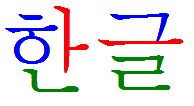han geul

Every hangeul is made of letters.
These are called "Jamo", 자모 in Korean.

Korean syllables are written in such a way that they can all be confined within a square. The reason is simple: before the invention of this new writing system, Korean was written using Chinese characters, each character being confined in a square.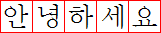One square equals one hangeul

The structure of Hangul: initial(s), vowel(s) and final(s)

Each hangeul is made of initial (consonants), vowels and sometimes finals (also consonants).

Due to the shape of certain vowels (one can speak of "vertical" or "horizontal" vowels) they are placed either to the right, or below the initial consonant.

Examples of vertical vowels:

(a), (i)

Examples of horizontal vowels:

(o), (u)

These vowels can be associated with consonants, such as (n), to form the following hangeul: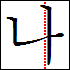(na), or (ni), with a vertical division, in which case the square is divided vertically between the two letters: between half and two thirds for the consonant, the rest for the vowel.(no), or (nu), with a horizontal cut, in which case the square is divided horizontally between two letters, about half the top to the consonant, the rest for the vowel.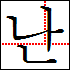Considering that Korean does not only consist of open syllables, but also closed syllables, many Hangeul include (at least) a final. No matter where the vowel is placed in regard to the initial, the final is always written below other jamo in the bottom third. For instance, hangul (nan) or (nun).

Diphthongs in Korean

Nonetheless diphthongs exist in Korean. In this case, the first vowel (which is necessarily a "horizontal" vowel) is first written below the initial (as in ), and followed by the second vowel (which is necessarily a "vertical" vowel).

With the letters studied in the previous examples, we have (let's not worry about the pronunciation right now, and leave that for the next lesson):

(n) + (o) + (a) =>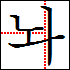(n) + (u) + (i) =>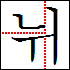Syllables including diphthongs can obviously also take finals. For example:

(n) + (o) + (a) + (n) =>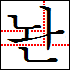For now, let's not pay attention to whether these phonemes have meanings or not and leave vocabulary to the next lessons ;-)